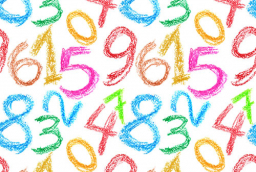# Significant 71974

The difference of three times the two numbers is 33. The first number is 11, more significant than the second number. What is the value of the second number?

b = n

### Step-by-step explanation:Did you find an error or inaccuracy? Feel free to write us. Thank you!

Tips for related online calculators
Do you have a linear equation or system of equations and looking for its solution? Or do you have a quadratic equation?